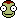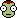# Fiddling with orbital equations

## Recommended Posts

Hi Everyone,

I'm having geeky fun with my HP-50g calculator, keying in all sorts of equations found on the Wiki pages. I know almost nothing in algebra/mathematics, but isn't KSP a nice place to learn?

I'm on Entroper's Basic Orbiting (Math) Wiki page and there's something I don't get. If I know the altitudes of my apo- and periapsis, I can solve for the orbital speeds at the apsides with the orbital speed equation

• velocity = body_radius * sqrt( 9.80665 / ( body_radius + altitude ) )

which would yield one velocity for the apo- and another for the periapsis. Right? Now, further down the tutorial page, there are equations useful to solve for numbers in elliptical orbits, and they use a variable l (lowercase L) for the angular momentum. It is said that "l = m * r * v at apoapsis or periapsis", where I understand that m means the orbiting vehicle's mass, r its orbit radius (body radius + altitude) and v its orbital velocity. It says "at apoapsis or periapsis", so I understand value of variable l should be equal whether it is calculated at apo- or periapsis, but, using the two values for v found using the velocity equation discussed above, I don't get equal numbers.

Are they not supposed to be equal? What am I not understanding?

Edited by Kebral
Trying to be more accurate, language-wise
##### Share on other sites

1 hour ago, Kebral said:

It says "at apoapsis or periapsis", so I understand value of variable l should be equal whether it is calculated at apo- or periapsis

Correct.  The angular momentum stays constant, as long as the orbiting body isn't affected by some other force such as rocket thrust.

1 hour ago, Kebral said:

but, using the two values for v found using the velocity equation discussed above, I don't get equal numbers.

Then something has gone off the rails somewhere.A few things:

• Make sure you're using the correct equation.  What you really want for calculating these velocities for an elliptical orbit is the vis-viva equation.  Try giving that a whirl.
• Don't mix apples and oranges.  The relevant "radius" numbers you want should be distance from center of body, not height above its surface.  (If you're applying stuff to KSP in game, beware-- the game displays Ap and Pe as height above surface, which muddies the waters considerably.  To preserve your sanity, best to convert to actual radii before cranking through the numbers.)

Do you have a specific example to play with, by way of illustration?  i.e. "I have an orbit around a body of such-and-such mass, with a periapsis of this and an apoapsis of that."

##### Share on other sites

To echo @Snark, when I wanted to do calculations on interplanetary transfers for my blog, I just used the the vis-visa equation.

I really should update that post with nicely formatted math.

##### Share on other sites

It's a few more fractions and exponents and parenthesis for my brain to process... I'll get back when I've played around with the vis-visa equation.

Yes, I understand r is expressed as orbit radius, and not vessel altitude, that's why I write body_radius + vessel_altitude. Still I must have goofed some place.

No particular example here, just pure theory. I'm trying to get results to match first before getting really confused applying them in-game...##### Share on other sites

9 hours ago, Kebral said:

velocity = body_radius * sqrt( 9.80665 / ( body_radius + altitude ) )

This equation is correct for circular orbits only. It is not correct for apsides of the elliptical orbit. Also, be careful with units. The 9.8 in this equation is surface gravity in m/s2, which locks you into using meters for body radius and altitude. Note also that this is equivalent to a more conventional form v = sqrt(GM/r)

##### Share on other sites

1 hour ago, K^2 said:

This equation is correct for circular orbits only. It is not correct for apsides of the elliptical orbit.

Ha! There it is. Using the Vis-viva equation, angular momentum is now constant! I get the same values for apo- and periapsis! Nice. Thanks a lot. The strange satisfaction of math...

Very nice blog page Mad Rocket Scientist. Thanks for that too.

Edited by Kebral
Typo
##### Share on other sites

9 hours ago, Kebral said:

Ha! There it is. Using the Vis-viva equation, angular momentum is now constant! I get the same values for apo- and periapsis! Nice. Thanks a lot. The strange satisfaction of math...

Very nice blog page Mad Rocket Scientist. Thanks for that too.﻿

## Join the conversation

You can post now and register later. If you have an account, sign in now to post with your account.
Note: Your post will require moderator approval before it will be visible.×   Pasted as rich text.   Paste as plain text instead

Only 75 emoji are allowed.